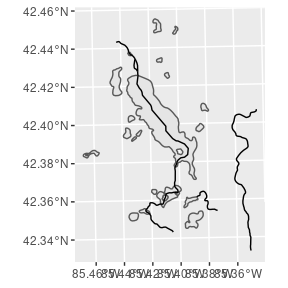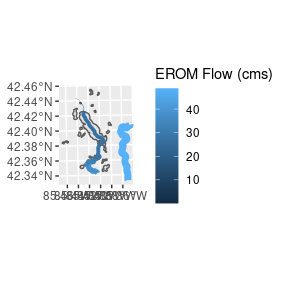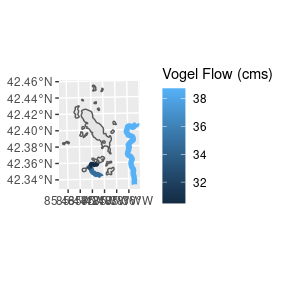# Querying flow information

#### 2021-06-06

Let’s get some flow data for the NHDPlus subset included with nhdR package. Our goal is to adjust the color and size of each reach by their average flow.

library(nhdR)
library(dplyr)
library(ggplot2)
library(sf)
ggplot() +
geom_sf(data = gull$sp$NHDWaterbody) +
geom_sf(data = gull$sp$NHDFlowLine)gull_sf <- gull$sp$NHDFlowLine

NHDPlus includes two estimates of flow:

## Enhanced Unit Runoff Method (EROM)

eromflow  <- nhd_plus_load(4, "EROMExtension", "EROM_MA0001") %>%
filter(ComID %in% gull$sp$NHDFlowLine$COMID) %>% select(ComID, Q0001F) gull_sf <- left_join(gull_sf, eromflow, by = c("COMID" = "ComID")) ## Vogel Flow Estimation vogelflow <- nhd_plus_load(4, "VogelExtension", "vogelflow") %>% filter(COMID %in% gull$sp$NHDFlowLine$COMID,
MAFLOWV != -9999.00000)

gull_sf <- left_join(gull_sf, vogelflow, by = "COMID")

## Visualization

gull_sf <- dplyr::filter(gull_sf, !is.na(Q0001F))
gull_sf %>%
ggplot() +
geom_sf(data = gull$sp$NHDWaterbody) +
geom_sf(aes(color = gull_sf$Q0001F), size = gull_sf$Q0001F / 20) +
labs(color = "EROM Flow (cms)")
#> Warning: Use of gull_sf$Q0001F is discouraged. Use Q0001F instead.gull_sf <- filter(gull_sf, !is.na(MAFLOWV)) gull_sf %>% ggplot() + geom_sf(data = gull$sp$NHDWaterbody) + geom_sf(aes(color = gull_sf$MAFLOWV), size = gull_sf$MAFLOWV / 20) + labs(color = "Vogel Flow (cms)") #> Warning: Use of gull_sf$MAFLOWV is discouraged. Use MAFLOWV instead.